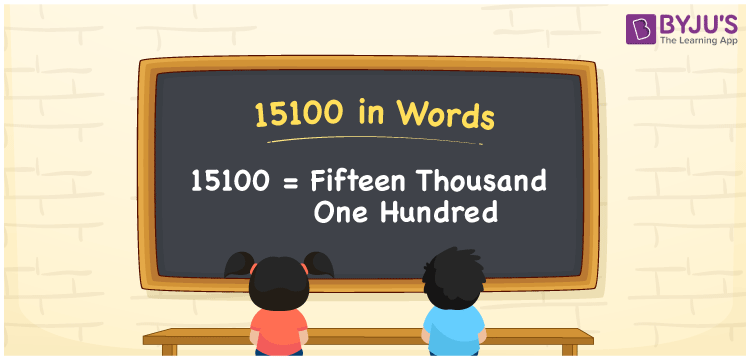# 15100 in Words

The number 15100 in words is “Fifteen Thousand One Hundred”. As we all know, 15100 is a cardinal number that represents the value or count of an object. To convert the number 15100 into words, use the place value table. If the cost of the mobile is Rs. 15100, then it can be written as “The cost of the mobile is Rs. Fifteen thousand one hundred”. Here is a detailed explanation of how to write the number 15100 in words.

 15100 in Words: Fifteen Thousand One Hundred Fifteen Thousand One Hundred in Numerical Form: 15100

## 15100 in English Words

15100 is written as follows in English words:## How to Write 15100 in Words?

With the help of the place value system, the number 15100 can be written in words. Below is a table that shows the place values for the number 15100.

 Ten-thousands Thousands Hundreds Tens Ones 1 5 1 0 0

The following is the expanded form of the number 15100.

= 1 × Ten thousand + 5 × Thousand + 1 × Hundred + 0 × Ten + 0 × One

= 1 × 10000 + 5 × 1000 + 1 × 100

= 10000 + 5000 + 100

= 15100

= Fifteen Thousand One Hundred

Hence, 15100 in words is Fifteen Thousand One Hundred.

Below is the interesting facts about the number 15100:

15100 in words – Fifteen thousand one hundred

Is 15100 an odd number? – No

Is 15100 an even number? – Yes

Is 15100 a perfect square number? – No

Is 15100 a perfect cube number? – No

Is 15100 a prime number? – No

Is 15100 a composite number? – Yes

## Frequently Asked Questions on 15100 in Words

Q1

### Write 15100 in words.

15100 in words is Fifteen Thousand One Hundred.

Q2

### Simplify 16000 – 900, and express in words.

Simplifying 16000 – 900, we get 15100. Hence, 15100 in words is Fifteen Thousand One Hundred.

Q3

### How to write fifteen thousand one hundred in numbers?

Fifteen Thousand One Hundred in numbers is 15100.

Q4

### What is the place value of 1 in the number 15100?

The place value of 1 in the number 15100 is 1х100 = 100
Q5

### Write the face value of 5 in the number 15100.

The face value of 5 in the number 15100 is 5 only.